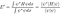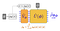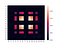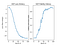Variational Quantum Thermalization and the Future of Quantum Thermodynamics

Quantum mechanics has had a profound impact on our understanding of the Universe and the level of technology we can build. Without knowledge of quantum mechanics, we would not have modern day electronics such as smartphones and laptops, lasers, fiber optics nor electric vehicle batteries to name just a few. We also know that the physics underlying the biochemical reactions and molecular biology which describes all living things in nature, from trees to neurons in our brains are fundamentally ruled by quantum mechanics. However, simulating quantum mechanical systems has proven to be one of the most difficult computational problems we’ve ever faced. One of the key issues at the heart of the matter is that somehow, nature is able to carry out a type of “bookkeeping” of quantum states that is well beyond our abilities to simulate with regular, classical computers. Suppose we want to store the full quantum state of an n-body quantum system. Storing the state would require 2^N classical bits. In order to keep track of a quantum system made up of only 200 electrons for example, a classical computer (the ones the world currently runs on) would need to store 2²⁰⁰ states. According to the US Department of Energy, planet Earth is made up of 2¹⁶⁶ atoms. So even if we turned the entire planet into a supercomputer where each atom acted as one classical bit, we wouldn’t be able to store the quantum state of 200 electrons. Our bodies are made of more than trillions of atoms each with one or more electrons in their electron shells. Somehow, somewhere, Nature keeps track of the quantum states of every electrons, photon and particle that comprise the Universe we live in. Note that this does not mean we can actually physically store 2²⁰⁰ classical bits of information in a quantum state of a 200-body quantum system. There is an upper bound of classical information that we can store known as Holevo’s bound, which states that we can only encode one bit of classical information for every quantum bit (qubit) we have.

In order to simulate nature therefore, given that it is inherently quantum mechanical, we need to circumvent the limitations that classical computers come with. In order to do this, Richard Feynman proposed in a 1982 paper that we need a computer that is also fundamentally based on quantum mechanical principles, in order to simulate quantum mechanical systems. In so doing so, he invented a new field of science known as Quantum Computing. It is only in the past decade that we have seen success in creating metaphorically small, noisy quantum computers prone to errors yet provably capable of processing quantum information. The first quantum algorithms meant to run on quantum computers as opposed to regular classical computers, were designed to run on idealized quantum computers that were fault-tolerant to errors and consisted of millions or billions of qubits. We are quite far away from realizing such machines with some of the best quantum computers in the world achieving implementations of as many as 53 qubits. The physical limitations of today’s quantum computing devices has sparked an era of quantum computing known as Noisy Intermediate Scale Quantum (NISQ), where NISQ era algorithms are meant to run on devices that exist today in order to produce useful results which don’t depend on perfect, large-scale quantum computers. One of the most successful NISQ algorithms has been the Variational Quantum Eigensolver (VQE). VQE is an ingenious method of approximating the ground state for a given Hamiltonian. It is based on the Rayleigh-Ritz method of approximating the lowest eigenvalue of the Hamiltonian we are interested in by using parametrized trial wave functions, then varying the parameters until the expectation value of the Hamiltonian operator is minimized.Rayleigh-Ritz method minimizes the energy of the trial wave function with respect to a given Hamiltonian provided each wave function is normalized. One can classically optimize this set of parameters which parametrize our trial wave function, such that the loss function represented by the free energy of the system is minimized.

Since we vary the parameters classically and only calculate the expectation value quantum mechanically on a quantum computing device, it would be computationally intractable and inefficient to traverse the entire Hilbert space of most of the systems that we are interested in. Thus, we choose to vary the parameters over a subspace of the Hilbert space instead, and our choice of subspace is called the ansatz. In the quantum computing regime, our ansatz is built using parametrized unitary quantum gates. The most common ansatz in quantum computing for simulating molecules and other quantum systems is the Unitary Coupled Cluster ansatz and the Bethe ansatz. Recall that the equation for both the classical and quantum free energy of a system is F = -ST + E where S is the entropy, T is the temperature and E is the average energy of the system. Because VQE classically optimizes a given set of parameters in the ansatz and the Von Neumann entropy of a pure state is zero, the optimization problem we are solving is one of variationally minimizing the free energy where F = E. Thus, the free energy is the loss function in the VQE regime.Loss function for the VQT, parametrized by θ and ϕ. Source: https://arxiv.org/pdf/1910.02071.pdf

Variational Quantum Thermalization (VQT) is a powerful NISQ algorithm that generalizes the VQE concept to mixed states rather than pure states. For a given density matrix representing the thermal state of a mixed state quantum system, the VQT variationally creates a modular Hamiltonian representation which recovers the underlying pure states and their associated classical probabilities, allowing for the reconstruction of the target thermal state density matrix we are interested in. In other words, minimization of the full quantum thermodynamic free energy function is realized, which implies that we parametrize the classical probabilities used in creating the density matrix and thus the Von Neumann entropy of the system of interest. θ parametrizes the classical probability distribution of the pure states we are trying to learn and generate, while ϕ parametrizes the ansatz for creating the pure states themselves. Thus our full circuit becomes the following:An abstraction of the full quantum circuit used to implement VQT. We prepare an initial density matrix parametrized by θ and ϕ. We then classically vary both parameters in order to minimize the loss function, using the quantum computer only for calculating the expectation value given the parametrized pure state that is being output based on some classical probability distribution pθ(Ψ). Source: https://arxiv.org/pdf/1910.02071.pdf

For a given Hamiltonian, a target thermal state and a target temperature 1/β, the VQT applies a series of single qubit and 2-qubit rotation gates parametrized by ϕ, applied to input pure states that are output based on a given classical probability distribution parametrized by θ. As a result, it is not just the choice of ansatz that matters, but the probability function that also matters. Further, the seminal paper defining VQT by Verdon et al. (2019) utilizes energy based models parameterized over a hypothesis space of energy functions, as is the case in classical machine learning energy based models, but it is instead generalized to the quantum regime. In every run and iteration of the VQT algorithm, we generate pure states based on the probability function of our choice, applying the ϕ parametrized quantum gates and measuring <Ψ|U†(ϕ)HU(ϕ)|Ψ>. After every iteration, a classical optimizer is used to vary the parameters θ and ϕ. Remember, the Von Neumann entropy is invariant under unitary action, so the entropy of U(ϕ)ρ(θ)U†(ϕ) is equal to the Von Neumann entropy of ρ(θ). Thus, the loss function of the VQT is minimized when U(ϕ)ρ(θ)U†(ϕ) is equal to the density matrix of the thermal state.

I implemented the VQT algorithm for the 2-Dimensional Quantum XY model, which is equivalent to the 2D Heisenberg XYZ model only with the coupling between Z components turned off. Below is a picture of the target state of the model that we are trying to learn the pure states and their associated probability distribution of.Visualization of the target state density matrix for the 2D XY model.

The algorithm uses a factorized latent representation in this case (though we could have also used one that isn’t). Meaning that the overall trial density matrix can be represented as singular one-qubit parametrized density matrices with their tensors taken sequentially together. This is only possible when the parametrized classical probability distribution is independent for each qubit. In this case, our choice of probability distribution is the Bernoulli distribution.Loss History and Fidelity of the VQT Algorithm for the 2D XY model for 100 epochs.

The algorithm runs for 100 epochs, ending with an overall fidelity (difference between our predicted Density matrix and the Thermal State Density matrix) of 0.9216441677811639 or ~92% fidelity. I printed out a snapshot of the density matrix after each epoch and turned it into a GIF animation so that we can visualize how the predicted density matrix evolves over time.

As we can see, the VQT does an amazing job at generating an approximation of the pure states and their probability distributions for the thermal state of the Quantum 2D XY Heisenberg Model. This algorithm is an example of how quantum computing can be applied to study quantum thermodynamics, the field where thermodynamics and quantum mechanics intersect. Quantum thermodynamics is sometimes referred to as the “Toddler” of physics. There is so much to learn and sort out when it comes to understanding how thermodynamics works at the quantum scale.

The rewards for learning it however could be quite interesting. Thermodynamics when it was first conceived was based on our understanding of the classical world, as it related to macroscopic engines and so on. Quantum thermodynamics applies to how thermodynamic laws behave at the quantum scale, and how those laws scale up and become classical at macroscopic scales. One of the most interesting applications of this field is the idea of building single-ion engines and in general quantum-scale machine systems. I believe mastery of this field and the necessary engineering will allow us to develop quantum-mechanical mechanistic systems from the ground up, such that we can develop engines, actuators and machines whose parts are built at atomic scales. I believe the emergent phenomena of these systems will resemble biological systems, so that we can create synthetic lifeforms with intelligence capabilities going beyond what our current hardware-software combination allows. If such a technology is ever realized, we could build quantum scale robotic-biological systems that interface with our cells, neurons, organelles and so on such that we can repair ourselves instantly whenever new diseases or bodily harm happens to us. Another application of this technology would be the ability to undergo synthetic metamorphosis so that our bodies are suited perfectly to live underwater, on the moon, or on other planets whose environments typically would not be conducive for life.

Hopefully this article shed some light on what the Variational Quantum Thermalizer algorithm is and also what the future of quantum thermodynamics might hold for us.

Yours Truly,